# 3. Consider the function f(x,y) = 4 + 2x - 3y - x2 + 2y2 -...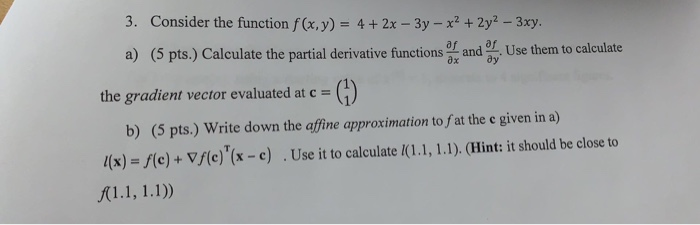3. Consider the function f(x,y) = 4 + 2x - 3y - x2 + 2y2 - 3xy. a) (5 pts.) Calculate the partial derivative functions, and use them to calculate the gradient vector evaluated at c = b) (5 pts.) Write down the affine approximation to at the e given in a) /(x) = f(c)+ Vf(e)'(x - c) . Use it to calculate (1.1, 1.1). (Hint: it should be close to f(1.1, 1.1))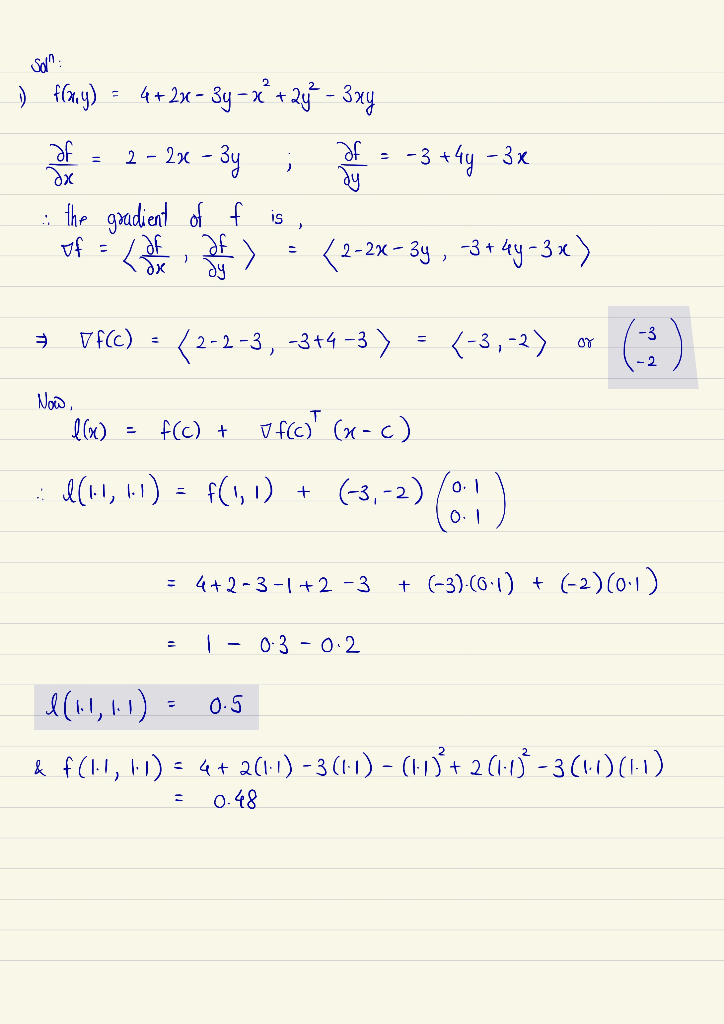#### Earn Coin

Coins can be redeemed for fabulous gifts.

Similar Homework Help Questions
• ### cense. This license can only be 5. Given f (x,y) = 12 + x2 + 2y2...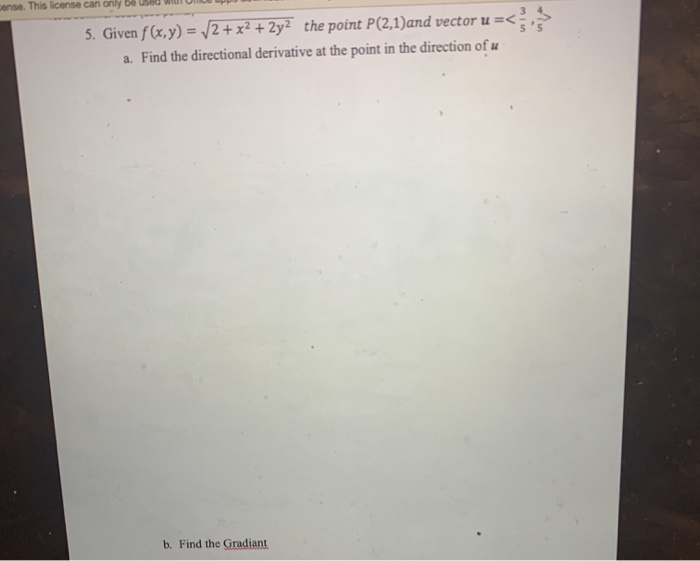cense. This license can only be 5. Given f (x,y) = 12 + x2 + 2y2 the point P(2,1)and vector u =< a. Find the directional derivative at the point in the direction of u b. Find the Gradiant

• ### All of 10 questions, please. 1. Find and classify all the critical points of the function. f(x,y) - x2(y - 2) - y2 » 2. Evaluate the integral. 3. Determine the volume of the solid that is inside the...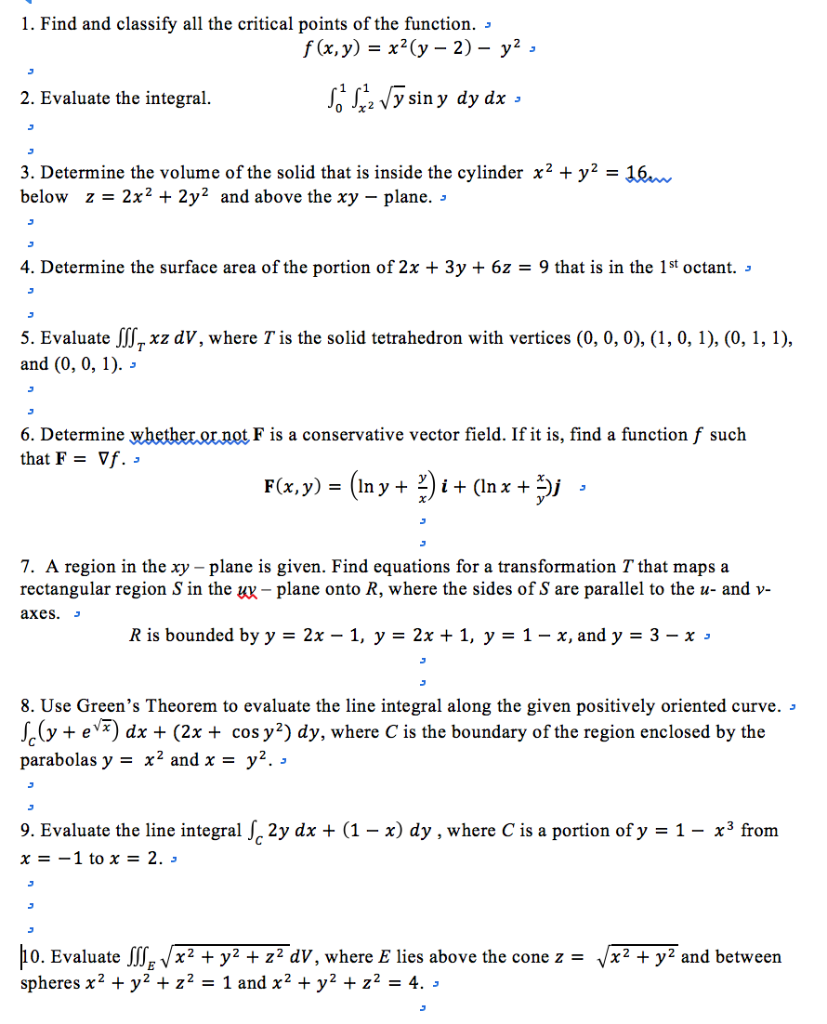All of 10 questions, please. 1. Find and classify all the critical points of the function. f(x,y) - x2(y - 2) - y2 » 2. Evaluate the integral. 3. Determine the volume of the solid that is inside the cylinder x2 + y2- 16 below z-2x2 + 2y2 and above the xy - plane. 4. Determine the surface area of the portion of 2x + 3y + 6z - 9 that is in the 1st octant. » 5. Evaluate JSxz...

• ### Consider the vector field F(x, y, z) -(z,2x, 3y) and the surface z- /9 - x2 -y2 (an upper hemisph...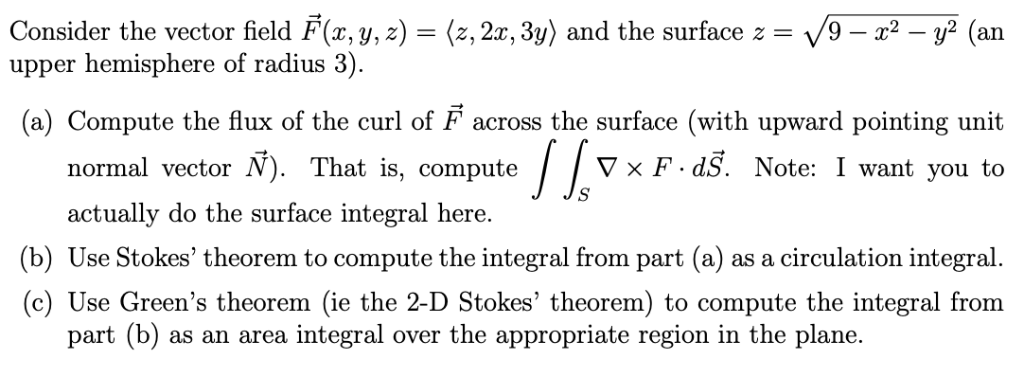Consider the vector field F(x, y, z) -(z,2x, 3y) and the surface z- /9 - x2 -y2 (an upper hemisphere of radius 3). (a) Compute the flux of the curl of F across the surface (with upward pointing unit normal vector N). That is, compute actually do the surface integral here. V x F dS. Note: I want you to b) Use Stokes' theorem to compute the integral from part (a) as a circulation integral (c) Use Green's theorem (ie...

• ### 5. Consider the function f: R -> R given by f (x, y) := e°+v* _...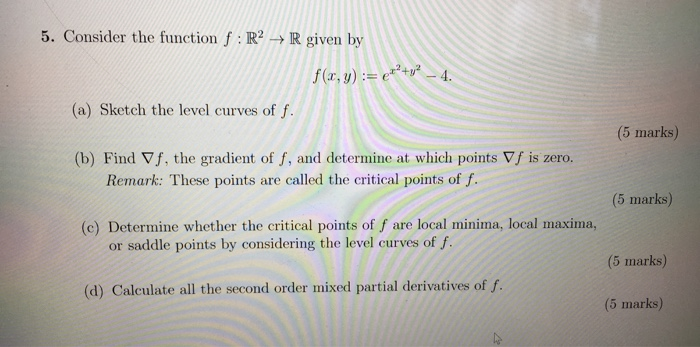5. Consider the function f: R -> R given by f (x, y) := e°+v* _ 4. (a) Sketch the level curves of f. (5 marks) (b) Find Vf, the gradient of f, and determine at which points Vf is zero. Remark: These points are called the critical points of f (5 marks) (c) Determine whether the critical points of f are local minima, local maxima, or saddle points by considering the level curves of f. (5 marks) (d) Calculate...

• ### Find the directional derivative D−→ u f(x,y) of the function f(x,y) = x2 + 3xy +...

Find the directional derivative D−→ u f(x,y) of the function f(x,y) = x2 + 3xy + y3 where →− u is the unit vector given by angle θ = π 4. What is D−→ u f(1,1)?

• ### Answer the following questions using the function -3y f(x,y) = 2y2 + 1 Plot fíx, y)...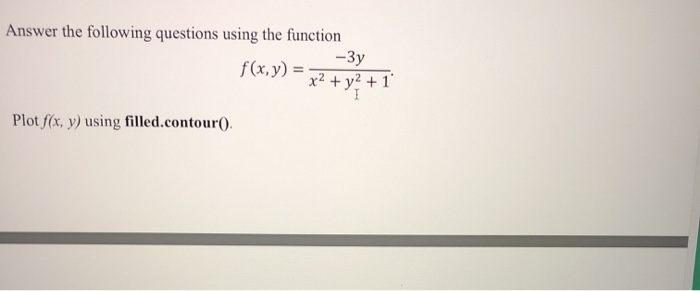Answer the following questions using the function -3y f(x,y) = 2y2 + 1 Plot fíx, y) using filled.contour) Please include code for ths Buestion Thanks Answer the following questions using the function -3y f(x,y) = 2y2 + 1 Plot fíx, y) using filled.contour) Please include code for ths Buestion Thanks

• ### Question 12 1 pts Let f(x, y) = 2x – y + x2 + y4. Which...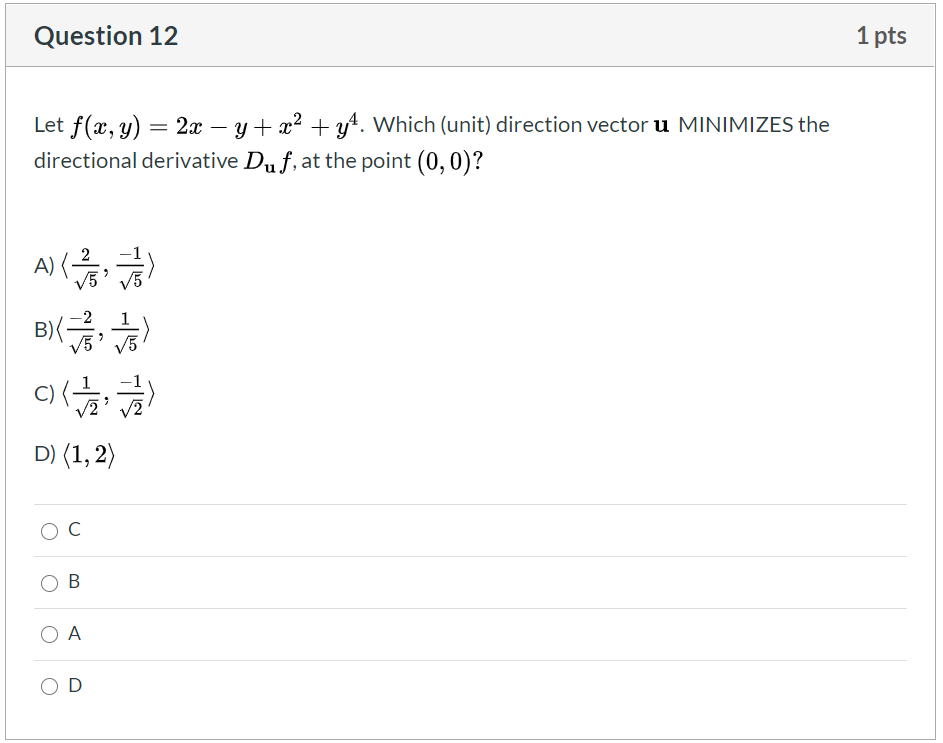Question 12 1 pts Let f(x, y) = 2x – y + x2 + y4. Which (unit) direction vector u MINIMIZES the directional derivative Duf, at the point (0,0)? ANG 1 B)(- 2 V5 C) < tai D) (1, 2) OC B OA D

• ### Find the derivative of the function f(x) = (2x-5)4​(x2+x+1)5

Find the derivative of the function f(x) = (2x-5)4(x2+x+1)5

• ### Exam 2018s1] Consider the function f R2 R, defined by f(x,y) =12y + 3y-2 (a) Find the first-order Taylor approximation at the point Xo-(1,-2) and use it to find an approximate value for f(1.1,-2.1 (b...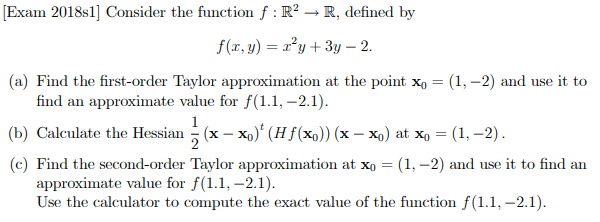Exam 2018s1] Consider the function f R2 R, defined by f(x,y) =12y + 3y-2 (a) Find the first-order Taylor approximation at the point Xo-(1,-2) and use it to find an approximate value for f(1.1,-2.1 (b) Calculate the Hessian 1 (x-4)' (Hr(%)) (x-%) at X-(1-2) c) Find the second-order Taylor approximation at xo- (1,-2) and use it to find an approximate value for f(1.1,-2.1 Use the calculator to compute the exact value of the function f(11,-2.1) Exam 2018s1] Consider the function...

• ### Questions A and E (b) f(x, y) = 2.2-2y2-6x + 8y + 3 (c) f(z, y)=x2...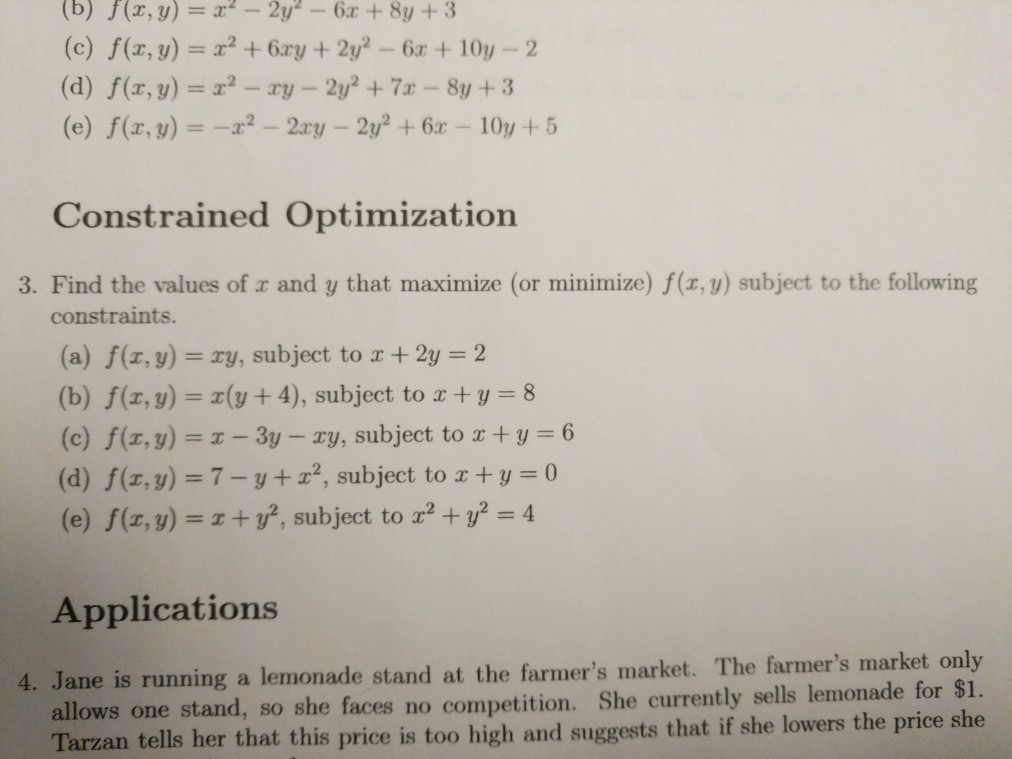Questions A and E (b) f(x, y) = 2.2-2y2-6x + 8y + 3 (c) f(z, y)=x2 + 6ry + 2/-6x +10y-2 Constrained Optimization 3. Find the values of r and y that maximize (or minimize) f(x,y) subject to the following constraints (a) f(z, y) = ry, subject to x + 2y = 2 (b) f(z, y)y+4), subject to z+y 8 (c) f(x,y) = x-3y-xy, subject to x + y = 6 (d) f(x, y)-7-y 2, subject to z ty 0...